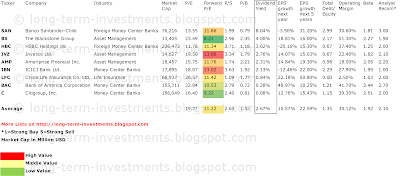## Pages

### 9 Financial Dividend Stocks With +15% Expected 5Y Earnings Growth At Cheap Prices

Financial dividend stocks with high beta and growth ratios and a low valuation originally published at long-term-investments.blogspot.com. The financial sector still suffers under the aftermath effects of the Lehman crises and the current low interest environment. But some financial stocks are back to pay good dividends to shareholders.

In my today’s screen about high beta dividend financial stocks I needed to implement some additional criteria in order to limit the huge screen amounts. You can see the detailed limitations here:

- Market Capitalization over USD 10 billion
- Positive Dividend Yield
- Forward P/E below 15
- Beta Ratio over one
- 5-Year Future Earnings Growth Rate Over 15% yearly

Only nine stocks fulfilled these strong criteria. Seven of them have a current buy or better rating by brokerage firms and one high yield stocks is part of the results.

Here are the highest yielding results:
If you would like to receive more dividend stock ideas and the free Dividend Weekly, you should subscribe to my free e-mail list. Alternatively, you can follow me on Facebook or Twitter.

Banco Santander (NYSE:SAN) has a market capitalization of \$80.71 billion. The company employs 186,763 people, generates revenue of \$78.750 billion and has a net income of \$3.967 billion. Banco Santander’s earnings before interest, taxes, depreciation and amortization (EBITDA) amounts to \$21.931 billion. The EBITDA margin is 27.84 percent (the operating margin is 7.68 percent and the net profit margin 6.44 percent).

Financial Analysis: The total debt represents 17.66 percent of Banco Santander’s assets and the total debt in relation to the equity amounts to 300.33 percent. Due to the financial situation, a return on equity of 2.93 percent was realized by Banco Santander. Twelve trailing months earnings per share reached a value of \$0.56. Last fiscal year, Banco Santander paid \$0.78 in the form of dividends to shareholders. Forward P/E: 11.86 at expected 5-Year annual earnings growth of 31.20 percent.

Market Valuation: Here are the price ratios of the company: The P/E ratio is 13.48, the P/S ratio is 1.36 and the P/B ratio is finally 0.81. The dividend yield amounts to 10.44 percent and the beta ratio has a value of 1.81.Long-Term Stock Price Chart Of Banco Santander (SAN)Long-Term Dividend Payment History of Banco Santander (SAN)Long-Term Dividend Yield History of Banco Santander (SAN)

Blackstone Group (NYSE:BX) has a market capitalization of \$12.96 billion. The company employs 1,780 people, generates revenue of \$4.019 billion and has a net income of \$829.88 million. Blackstone Group’s earnings before interest, taxes, depreciation and amortization (EBITDA) amounts to \$2.084 billion. The EBITDA margin is 51.86 percent (the operating margin is 18.88 percent and the net profit margin 20.65 percent).

Financial Analysis: The total debt represents 45.11 percent of Blackstone Group’s assets and the total debt in relation to the equity amounts to 238.74 percent. Due to the financial situation, a return on equity of 4.31 percent was realized by Blackstone Group. Twelve trailing months earnings per share reached a value of \$1.07. Last fiscal year, Blackstone Group paid \$0.52 in the form of dividends to shareholders. Forward P/E: 8.21 at expected 5-Year annual earnings growth of 16.0 percent.

Market Valuation: Here are the price ratios of the company: The P/E ratio is 21.44, the P/S ratio is 6.55 and the P/B ratio is finally 2.34. The dividend yield amounts to 4.56 percent and the beta ratio has a value of 2.27.Long-Term Stock Price Chart Of Blackstone Group (BX)Long-Term Dividend Payment History of Blackstone Group (BX)Long-Term Dividend Yield History of Blackstone Group (BX)

HSBC Holdings (NYSE:HBC) has a market capitalization of \$205.83 billion. The company employs 260,400 people, generates revenue of \$56.702 billion and has a net income of \$15.334 billion. HSBC Holdings’s earnings before interest, taxes, depreciation and amortization (EBITDA) amounts to \$24.018 billion. The EBITDA margin is 42.36 percent (the operating margin is 27.31 percent and the net profit margin 20.28 percent).

Financial Analysis: The total debt represents 10.32 percent of HSBC’s assets and the total debt in relation to the equity amounts to 158.61 percent. Due to the financial situation, a return on equity of 8.06 percent was realized by HSBC Holdings. Twelve trailing months earnings per share reached a value of \$4.14. Last fiscal year, HSBC Holdings paid \$2.25 in the form of dividends to shareholders. Forward P/E: 11.34 at expected 5-Year annual earnings growth of 15.30 percent.

Market Valuation: Here are the price ratios of the company: The P/E ratio is 13.35, the P/S ratio is 2.66 and the P/B ratio is finally 1.15. The dividend yield amounts to 4.31 percent and the beta ratio has a value of 1.25.Long-Term Stock Price Chart Of HSBC Holdings (HBC)Long-Term Dividend Payment History of HSBC Holdings (HBC)Long-Term Dividend Yield History of HSBC Holdings (HBC)

Take a closer look at the full list of cheap high beta financial dividend stocks with high earnings growth forecasts. The average P/E ratio amounts to 19.77 and forward P/E ratio is 11.22. The dividend yield has a value of 2.67 percent. Price to book ratio is 1.52 and price to sales ratio 2.63. The operating margin amounts to 30.12 percent and the beta ratio is 1.92. Stocks from the list have an average debt to equity ratio of 1.35.

Here is the full table with some fundamentals (TTM):High Beta Financial Dividend Stocks (Click to enlarge)